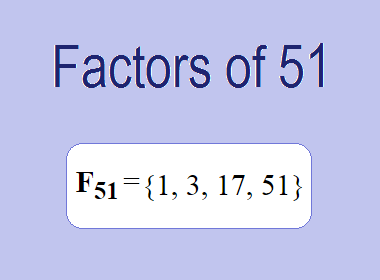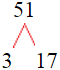# Factors of 51The factors of 51 are 1, 3, 17, and 51 i.e. F51 = {1, 3, 17, 51}. The factors of 51 are all the numbers that can divide 51 without leaving a remainder.

We can check if these numbers are factors of 51 by dividing 51 by each of them. If the result is a whole number, then the number is a factor of 51. Let's do this for each of the numbers listed above:

·        1 is a factor of 51 because 51 divided by 1 is 51.

·        3 is a factor of 51 because 51 divided by 3 is 17.

·        17 is a factor of 51 because 51 divided by 17 is 3.

·        51 is a factor of 51 because 51 divided by 51 is 1.

## How to Find Factors of 51?

1 and the number itself are the factors of every number. So, 1 and 51 are two factors of 51. To find the other factors of 51, we can start by dividing 51 by the numbers between 1 and 51. If we divide 51 by 2, we get a remainder of 1. Therefore, 2 is not a factor of 51. If we divide 51 by 3, we get a remainder of 0. Therefore, 3 is a factor of 51.

Next, we can check if 4 is a factor of 51. If we divide 51 by 4, we get a remainder of 3. Therefore, 4 is not a factor of 51. We can continue this process for all the possible factors of 51.

Through this process, we can find that the factors of 51 are 1, 3, 17, and 51. These are the only numbers that can divide 51 without leaving a remainder.

********************

********************

## Properties of the Factors of 51

The factors of 51 have some interesting properties. One of the properties is that the sum of the factors of 51 is equal to 72. We can see this by adding all the factors of 51 together:

1 + 3 + 17 + 51 = 72

Another property of the factors of 51 is that they are all odd numbers. This is because 51 is an odd number, and any even number cannot divide an odd number without leaving a remainder.

Another property of the factors of 51 is that the prime factors of 51 are 3 and 17.

## Applications of the Factors of 51

The factors of 51 have several applications in mathematics. One of the applications is in finding the highest common factor (HCF) of two or more numbers. The HCF is the largest factor that two or more numbers have in common. For example, to find the HCF of 51 and 34, we need to find the factors of both numbers and identify the largest factor they have in common. The factors of 51 are 1, 3, 17, and 51. The factors of 34 are 1, 2, 17, and 34. The largest factor that they have in common is 17. Therefore, the HCF of 51 and 34 is 17.

Another application of the factors of 51 is in prime factorization. Prime factorization is the process of expressing a number as the product of its prime factors. The prime factors of 51 are 3 and 17. We can express 51 as:

51 = 3 × 17

We can do prime factorization by division and factor tree method also. Here is the prime factorization of 51 by division method,51 = 3 × 17

Here is the prime factorization of 51 by the factor tree method,51 = 3 × 17

## Conclusion

The factors of 51 are the numbers that can divide 51 without leaving a remainder. The factors of 51 are 1, 3, 17, and 51. The factors of 51 have some interesting properties, such as being odd numbers and having a sum of 72. The factors of 51 have several applications in mathematics, such as finding the highest common factor and prime factorization.